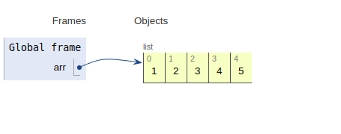# Python Program to find the sum of array

In this article, we will learn about the solution and approach to solve the given problem statement.

## Problem statement

Given an array as an input, we need to compute the sum of the given array.

Here we may follow the brute-force approach i.e. traversing over a list and adding each element to an empty sum variable. Finally, we display the value of the sum.

We can also perform an alternate approach using built-in sum function as discussed below.

## Example

# main
arr = [1,2,3,4,5]
ans = sum(arr,n)
print ('Sum of the array is ',ans)

## Output

15


All the variables & functions are declared in the global scope and are shown below.